International
Tables for
Crystallography
Volume B
Reciprocal space
Edited by U. Shmueli

International Tables for Crystallography (2006). Vol. B. ch. 2.1, p. 197   | 1 | 2 |

## Section 2.1.6.1. Distributions of sums and averages

U. Shmuelia* and A. J. C. Wilsonb

aSchool of Chemistry, Tel Aviv University, Tel Aviv 69 978, Israel, and bSt John's College, Cambridge, England
Correspondence e-mail:  ushmueli@post.tau.ac.il

#### 2.1.6.1. Distributions of sums and averages

| top | pdf |

In Section 2.1.2.1, it was shown that the average intensity of a sufficient number of reflections is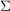[equation (2.1.2.4)]. When the number of reflections is not `sufficient', their mean value will show statistical fluctuations about; such statistical fluctuations are in addition to any systematic variation resulting from non-independence of atomic positions, as discussed in Sections 2.1.2.1–2.1.2.3. We thus need to consider the probability density functions of sums like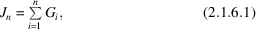and averages like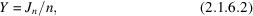where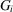is the intensity of the ith reflection. The probability density distributions are easily obtained from a property of gamma distributions: If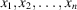are independent gamma-distributed variables with parameters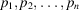, their sum is a gamma-distributed variable with parameter p equal to the sum of the parameters. The sum of n intensities drawn from an acentric distribution thus has the distribution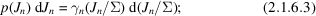the parameters of the variables added are all equal to unity, so that their sum is p. Similarly, the sum of n intensities drawn from a centric distribution has the distribution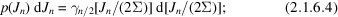each parameter has the value of one-half. The corresponding distributions of the averages of n intensities are then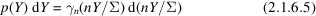for the acentric case, and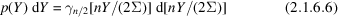for the centric. In both cases the expected value of Y isand the variances are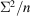and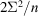, respectively, just as would be expected.